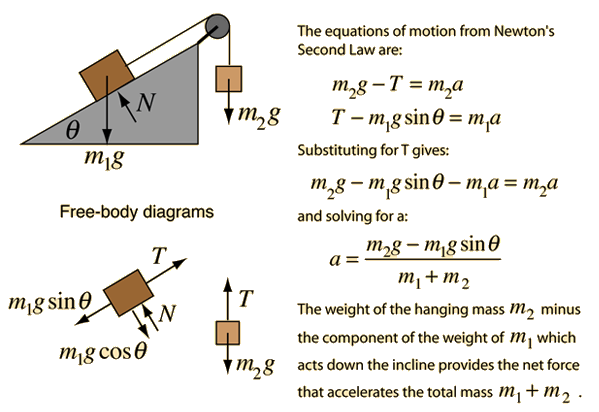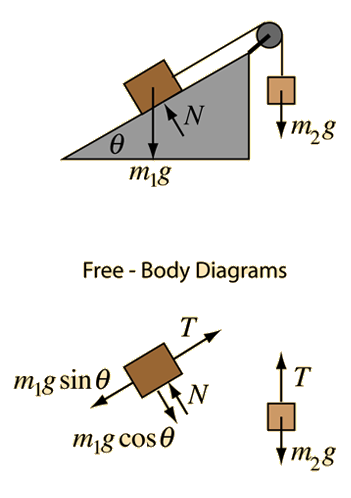# Inclined Pulley### Calculation

Index

Newton's laws

Standard mechanics problems

 HyperPhysics***** Mechanics ***** Newton's laws R Nave
Go Back

# Inclined PulleyNote that the tension in the rope is NOT equal to the weight of the hanging mass except in the special case of zero acceleration.
Application of Newton's second law to mass on incline with pulley.
Given an incline with angle degrees which has a mass of kg placed upon it. It is attached by a rope over a pulley to a mass of kg which hangs vertically. Taking downward as the positive direction for the hanging mass, the acceleration will be

### Acceleration =m/s²

With this acceleration, the tension in the rope will be
T= Newtons compared to the weight W = Newtons for the hanging mass. Exploring different values for the masses will allow you to show that the tension is less than the weight for downward accelerations and greater than the weight for upward accelerations when the net force on the hanging mass must be upward.